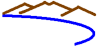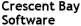Compiler technology => ExamplesOptimization technology: ExamplesWe have taken one small function and coded it several different ways to demonstrate the kinds of optimizations and transformations VAST can be used for. The goal is to give you some idea of the range of ways VAST can improve the code for a particular target. In practice, VAST can do several sorts of transformations at the same time, depending on the needs of the target system.

### Original

```#define L 160
#define N 40
short int in[L];
short int coef[N];
short int out[L];

void fir1()
{
int i,j;

for ( i=N; i < L; i++)
{
int sum = 0;

for (j = 1; j <= N; j++)
sum += (int)coef[j-1] * (int)in[i-j];

out[i] = ((sum + in[i]) >> 15);

}
}
```

### Normal inner unrolling: (standard stuff)

```  for ( i=N; i < LEN; i++)
{
int sum = 0;

for (j = 1; j <= N; j+=4) {
sum += (int)coef[j-1] * (int)in[i-j];
sum += (int)coef[j] * (int)in[i-j-1];
sum += (int)coef[j+1] * (int)in[i-j-2];
sum += (int)coef[j+2] * (int)in[i-j-3];
}

out[i] = ((sum + in[i]) >> 15);

}
```
If there are "left over" elements, then VAST generates cleanup code to process them.

### Reduction unrolled: (unrolled into separate accumulators)

```  for ( i=N; i < LEN; i++)
{
int sum = 0;

for (j = 1; j <= N; j+=4)
sum += (int)coef[j-1] * (int)in[i-j];
sum1 += (int)coef[j] * (int)in[i-j-1];
sum2 += (int)coef[j+1] * (int)in[i-j-2];
sum3 += (int)coef[j+2] * (int)in[i-j-3];
}
sum += sum1 + sum2 + sum3;
out[i] = ((sum + in[i]) >> 15);

}
```

### Unrolled Outer: (outer loop unrolled inside inner loop)

```  for ( i=N; i < L; i+=4)
{
sum = 0;
sum2 = 0;
sum3 = 0;
sum4 = 0;

#pragma no_array_dep  /* VAST can signal no dependence */
for (j = 1; j <= N; j++) {
sum += (int)coef[j-1] * (int)in[i-j];
sum2 += (int)coef[j-1] * (int)in[i+1-j];
sum3 += (int)coef[j-1] * (int)in[i+2-j];
sum4 += (int)coef[j-1] * (int)in[i+3-j];
}

out[i] = ((sum + in[i]) >> 15);
out[i+1] = ((sum2 + in[i+1]) >> 15);
out[i+2] = ((sum3 + in[i+2]) >> 15);
out[i+3] = ((sum4 + in[i+3]) >> 15);

}
```
Depending on the iteration counts, VAST may generate setup and/or cleanup code.

### Dependence Illumination: (data dependencies explicitly called out.)

```1  for ( i=N; i < L; i++)
2  {
3    int sum = 0;
4
5    for (j = 1; j <= N; j++)
6      sum = sum + (int)coef[j-1] * (int)in[i-j];
7
8    out[i] = ((sum + in[i]) >> 15);
9
10 }

Dependencies: (For example, could be added to IR nodes.)
Type  Line/Node1  Line/Node2  (loop/distance)
Flow  3L/sum      6R/sum      i/0
Flow  6L/sum      6R/sum      j/0
Flow  6L/sum      8R/sum      i/0
Input 6/coef      6/coef      i/?
Input 6/in        6/in        i/?

Live in:
Loop i: coef, in
Loop j: sum, coef, in

Live out:
Loop i: out
Loop j: sum
```
The actual method of passing detailed dependence information is generally customized to the needs of the target compiler. The compiler IR can be enhanced to allow nodes to have dependence arc lists attached, for example. There are simpler interfaces such as having VAST generate pragmas that inform the compiler when there are no loop-carried array dependencies, for example.

```  if ( (L-N)*N > 2000 ) {   /* If enough work */
parallel_launch(fir1_par1);  /* do in parallel */
else {
(scalar version)
}
```
(Actually, in this case L and N are known at compile time, so the choice as to which version of the loop to create would be done at compile time instead of run-time. The "threshold" varies based on the target system.)
```void fir1_par1()  /* new parallel routine */
{
int i,j,ip;
chunk = (L-N)/num_threads();  /* how much should I do? */
iend = L+(ip+1)*chunk;  /* where does my work end? */
if ( iend>L ) iend = L;
for ( i=N+ip*chunk; i < iend; i++) /* this thread's work */
{
int sum = 0;

for (j = 1; j <= N; j++)
sum += (int)coef[j-1] * (int)in[i-j];

out[i] = ((sum + in[i]) >> 15);

}
```

### Cache Prefetch: (can mark arrays for advance fetch)

```  for ( i2=N; i2 < L; i2+=8) {  /* process in strips */

cache_prefetch ( in[i2+8] );  /* get a future line */

for ( i=i2; i<8; i++)  /* process within strips */
{
int sum = 0;

for (j = 1; j <= N; j++)
sum += (int)coef[j-1] * (int)in[i-j];

out[i] = ((sum + in[i]) >> 15);

}
}
```
(VAST will insert startup/cleanup code for strip loop as needed.)

### Idiom recognition: (can replace code sequences with functions)

```  for ( i=N; i < L; i++)
{
int sum = 0;

sum = vsldot ( &coef, 1, &in[i-1], -1, N );

out[i] = ((sum + in[i]) >> 15);

}
```
VAST can recognize important idioms in a powerful and flexible way. This can allow critical operations to be coded as portable loop nests but executed as hand-coded functions.

### Vector SIMD:

For vector SIMD systems, the generated code can look like inline intrinsics that map to the underlying vector instructions, with a "strip" loop around the vector instructions and appropriate startup and cleanup code to deal with alignment issues and ragged vector lengths. VAST has a number of strategies for dealing with alignment issues for vector SIMD units, including runtime testing and on-the-fly alignment adjustment. This would generally be different for each vector SIMD target. In addition, VAST may combine vector SIMD code generation with other optimizations, for example unrolling an outer loop inside the inner vectorized loop to provide more potential instruction overlap. Without getting too specific, here is some example pseudo code for what VAST generates for certain vector SIMD targets:
```if ( iteration count is positive )
for ( all vectors )
fetch vector operands;
permute operands for alignment;
compute vector operations;
HomeContactLegal